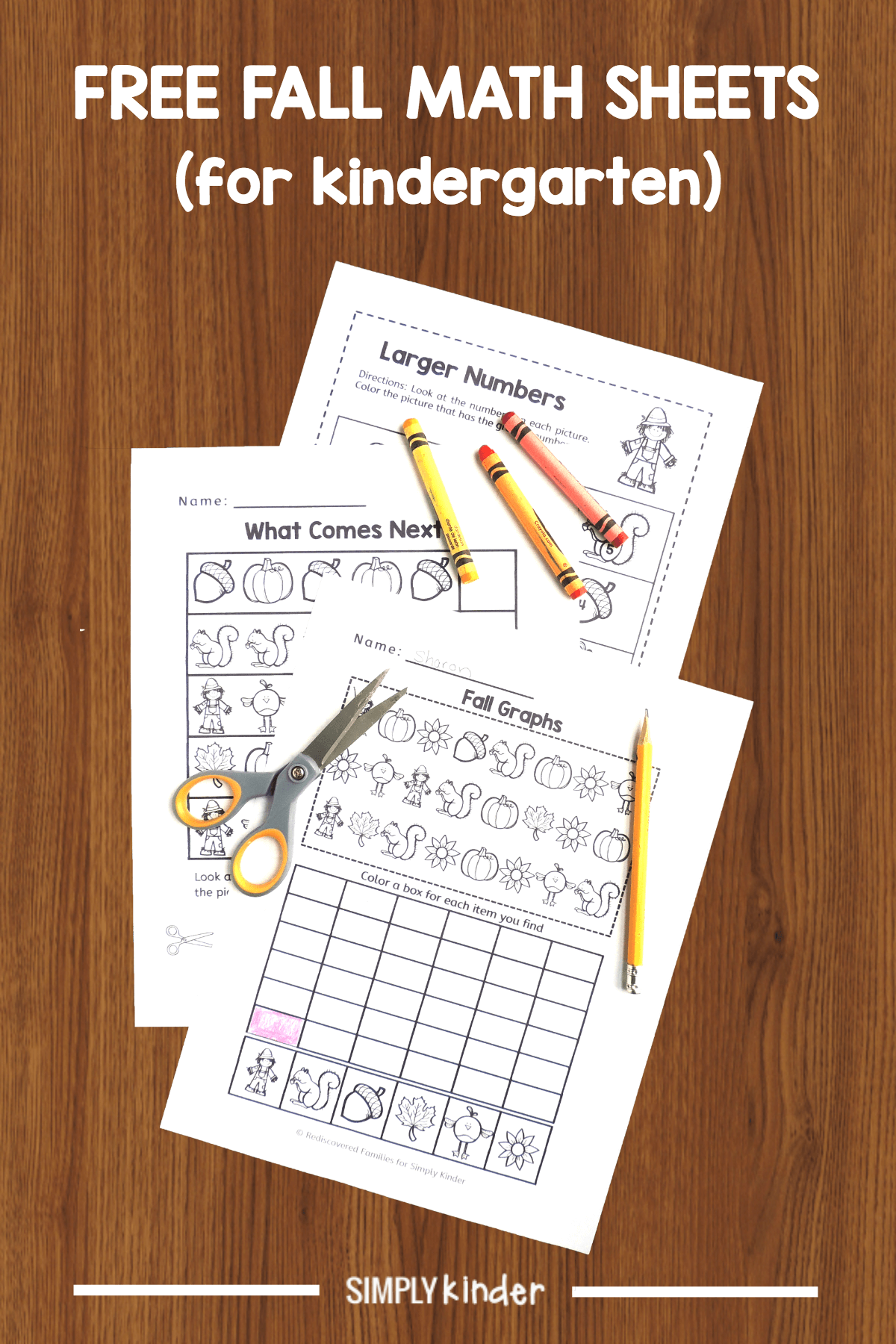Keep math prep to a minimum with these FREE Fall math worksheets. We’ve included pattern work, a simple graphing exercise, and comparing two numbers.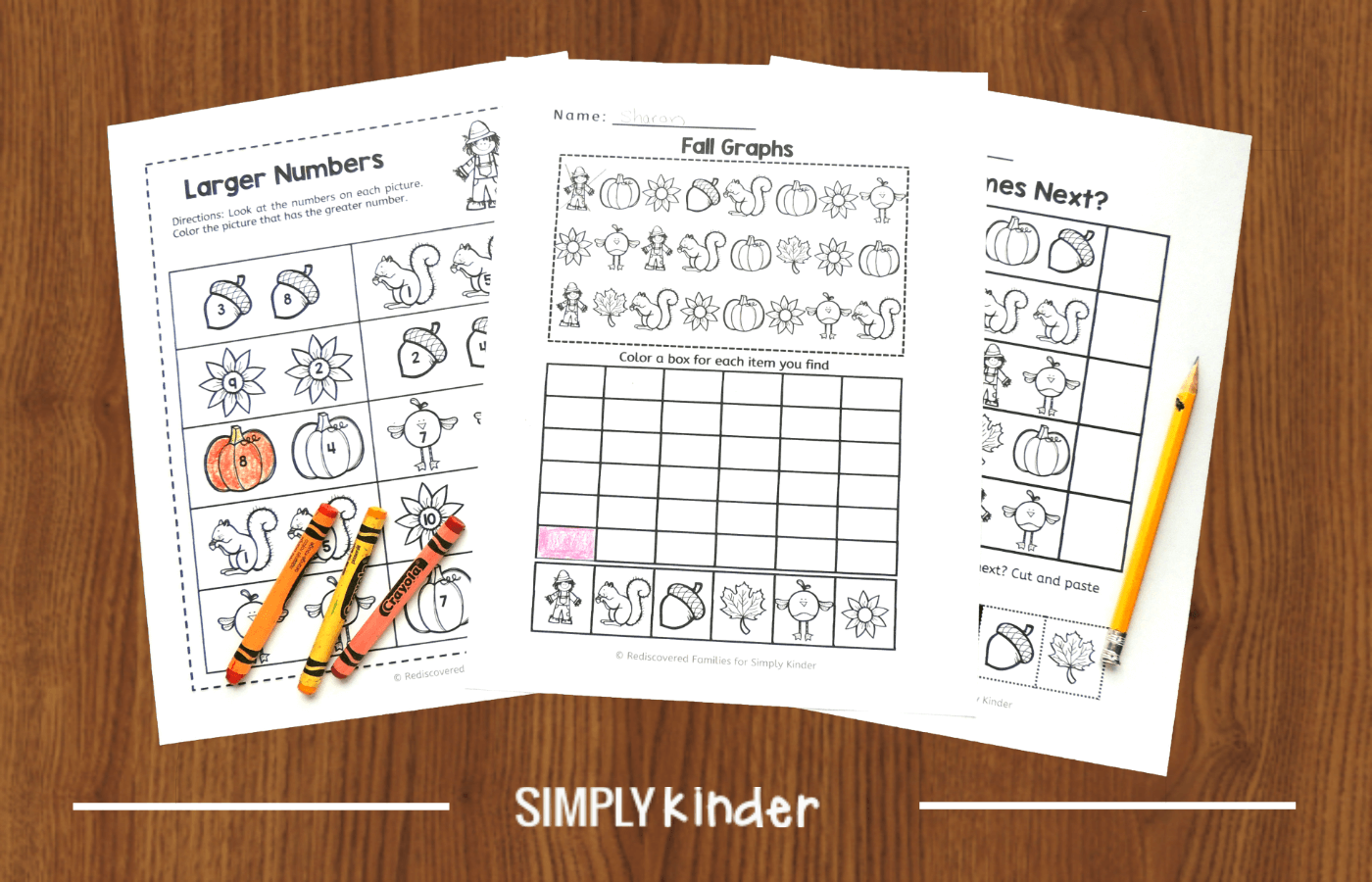Fall has definitely arrived in our part of the world. Everywhere you look there are signs that the seasons are changing, so why not incorporate them into your learning by using these FREE Fall Math Worksheets. This fun set will help your students practice some basic math skills.

### WHAT YOU WILL FIND IN THE FALL MATH WORKSHEETS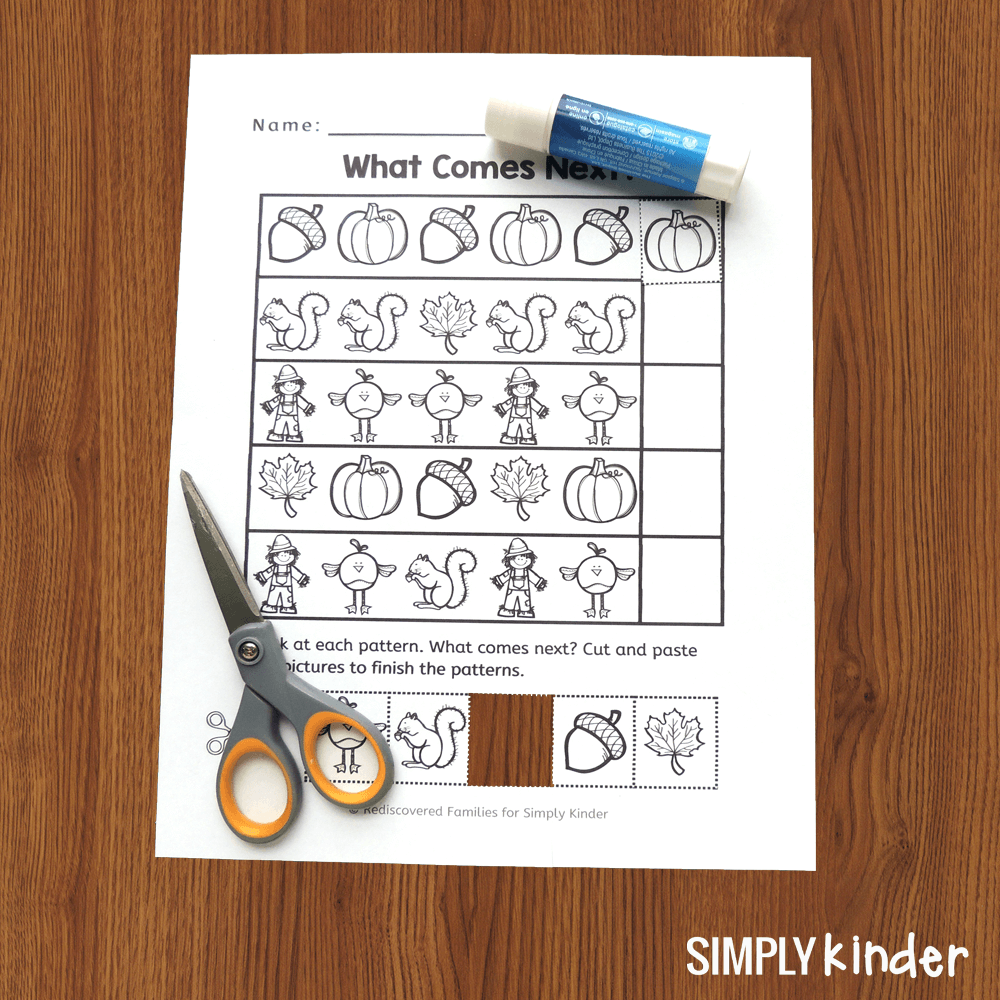1. The first worksheet helps children practice figuring out patterns. Encourage students to look at the object sequence in the boxes and figure out what should come next. The correct objects are featured below the boxes. Students will cut them out the pictures and glue the correct object into place.

Teacher tip: Children with the mathematical learning DYSCALCULIA will find it ALMOST IMPOSSIBLE to discern patterns. Learn more about Dyscalculia HERE.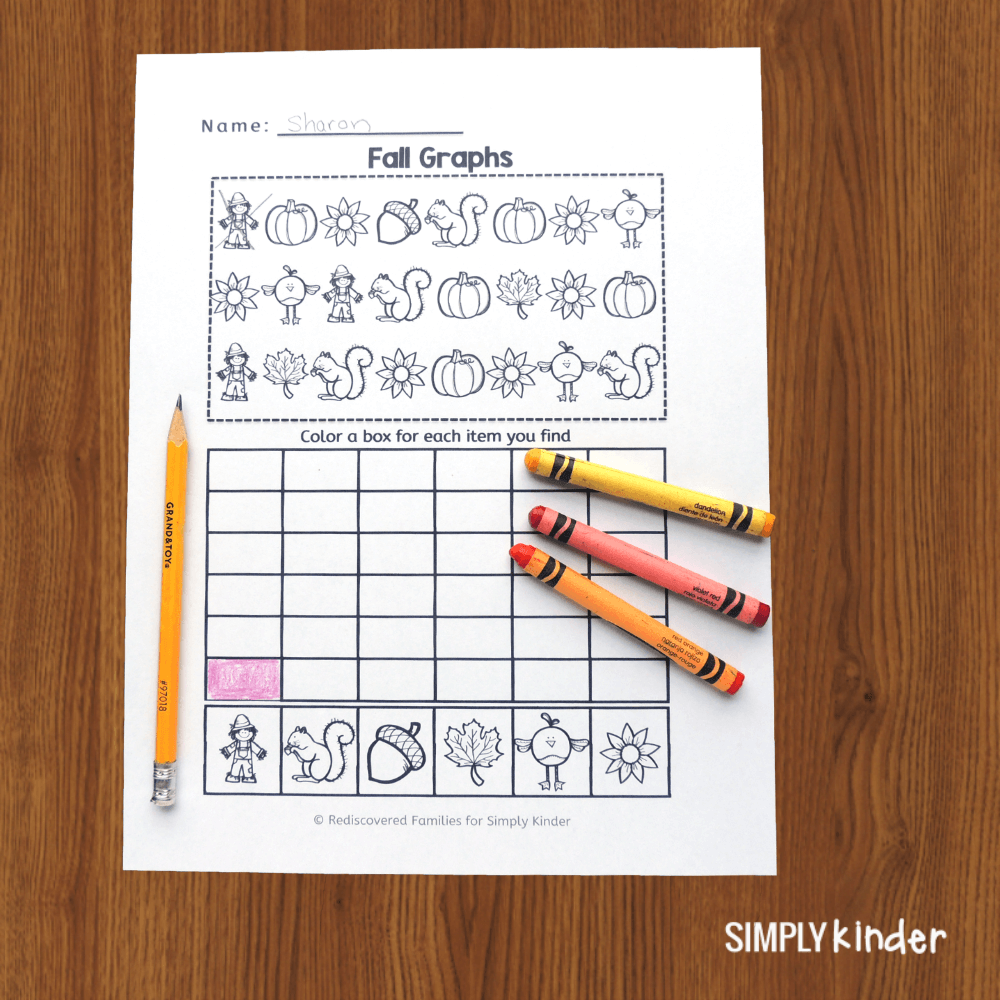2. The second worksheet invites students to create a simple graph. Invite students to count the fall objects and color in a graph square for every object they find. You might want to direct them to draw an X through the objects as they count them.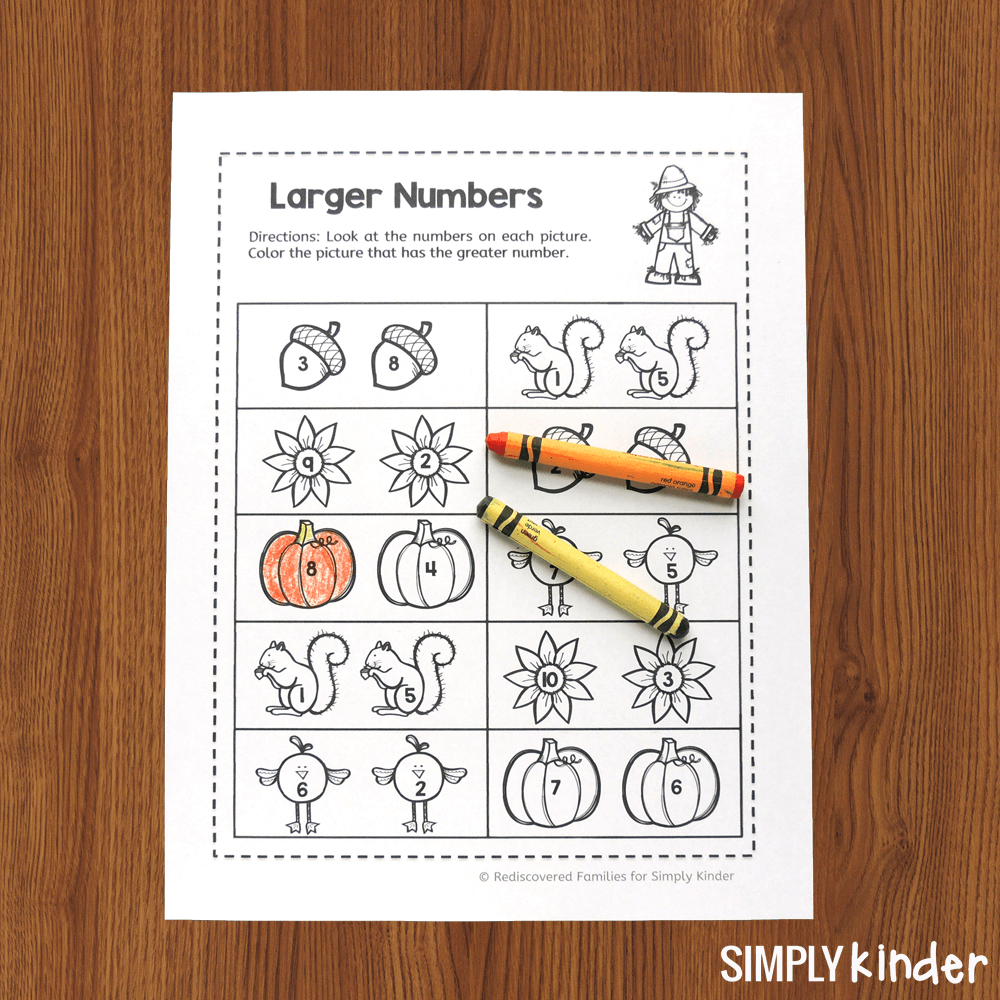3. The third worksheet asks the students to compare two numbers and pick the larger one. Students look at the numbers on each picture and then color the picture with the larger number.

### COMMON CORE STANDARDS

These printables will help your students practice basic math skills. They help with the following common core standards

• Compare two numbers between 1 and 10 presented as written numerals
• Understand the relationship between numbers and quantities.
• Classify objects into given categories; count the numbers of objects in each category and sort the categories by count.
• Look for and make use of structure (Mathematically proficient students look closely to discern a pattern or structure.)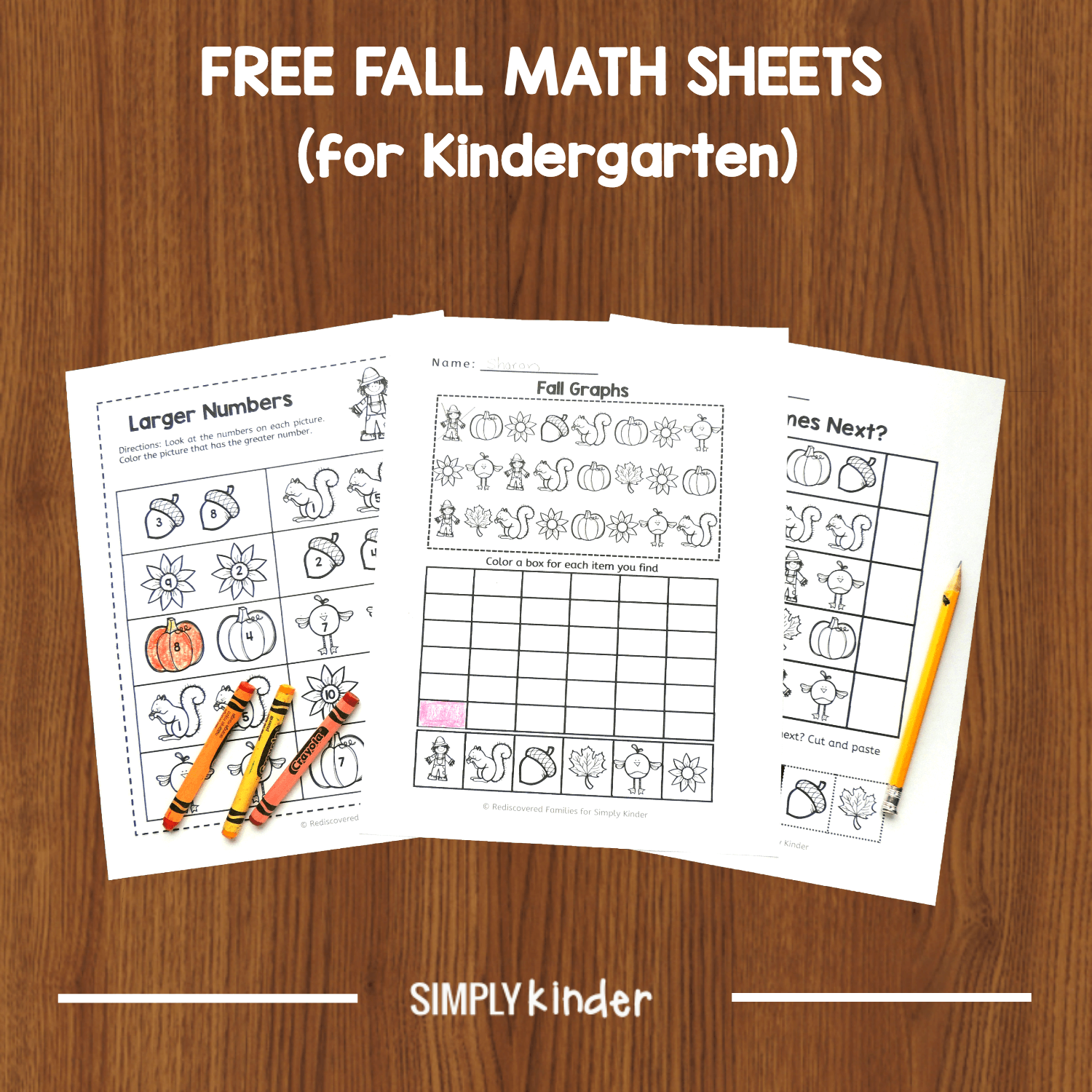### HOW TO USE YOUR FREE PRINTABLES

You can use these Fall math worksheets in the classroom or send them home as homework. They couldn’t be easier to prep. All you need to do is download them! Use for early finishers or as class activities. If you prefer, print and laminate the sheets, so they can be used multiple times. Just provide some dry erase markers.